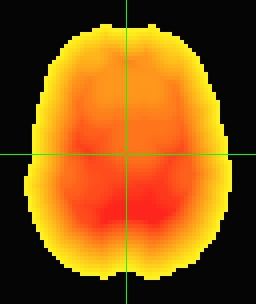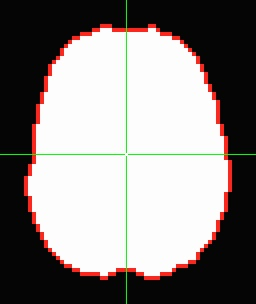I create a mask as ROI, now I want to add the edge for the mask. How can I do it?

Hi-

I don’t quite understand. Can you provide a bit more description? It is correct that you have a volume with a single ROI in it, and you just the edge of it?

There are different ways to do so; there is also some decision-making about what is meant by “edge”: are the voxels connected by any of voxel-faces or -nodes or -edges, or a subset of those? There are also various permutations depending on whether you have multiple ROIs per voxel (it sounds like that is not the case here).

The way I would start by doing this would be to calculate the depth of the ROI in terms of voxel counts:

``````
3dDepthMap \
-zeros_are_zero \
-ignore_voxdims \
-input DSET_ROI

``````

… and then use the following 3dcalc command to find nonzero places in the depth map below a certain value—using 1.2 here will keep a boundary connected by voxel-faces or voxel-edges:

``````
3dcalc \
-expr 'bool(a)*step(1.2-a)' \
-overwrite

``````

Those images are attached, showing the ROI as underlay, first with the depth calculation and then with the boundary calculation.

–ptHi，
Thank you so much for your reply. Actually, I created some masks as my ROI. And I give the masks different values. Then I use 3dcalc to compose these masks.like:3dcalc -a AN.nii -b VN.nii -c DMN.nii -d ECN.nii -expr ‘a+b+c+d’ -prefix Network.nii. The final result is the first picture.
Now what I want to do is add the edges for the mask. Then I will use SUMA to get a surface. So I want to add the edges to make the boundary between ROI clearer, like the second picture.

Paul has some cool solutions for showing edges in the volume, but you may be interested in showing contours on surface maps as described in this previous post: